# Cyberattacks Detection in IoT-based Smart City Network Traffic – Towards AI — The World’s Leading AI and Technology Publication

#### Author(s): Abhinav Dubey

Originally published on Towards AI the World’s Leading AI and Technology News and Media Company. If you are building an AI-related product or service, we invite you to consider becoming an AI sponsor. At Towards AI, we help scale AI and technology startups. Let us help you unleash your technology to the masses.

#### In this article, different machine learning and deep learning models have been used for the classification of cyberattacks such as DoS, Worms, Backdoor, and many more attacks from normal network traffic and network intrusion detection. UNSW-NB15 Dataset has been used to train the ML and DL models. You can find the complete code, trained models, plots, datasets, preprocessed files here on my GitHub account.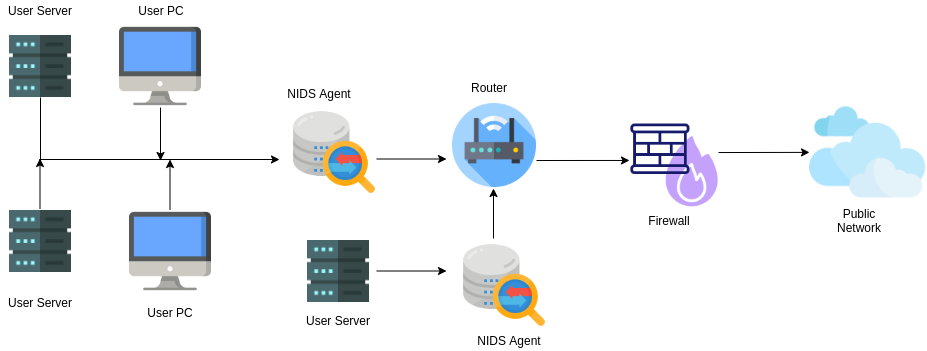Made using Draw.io

The whole idea of the Internet of Things is to extend the capability of the Internet beyond computers and smartphones to electronic, mechanical devices, sensors, etc. With the increasing number of use cases of IoT devices, security vulnerabilities have been also increased drastically.

Today IoT devices are used in fire systems, drones, smart homes, healthcare are just to name a few. You can imagine what a disaster it would be if someone with bad intent gets access to these systems. That’s why Network Intrusion Detection System (NIDS) is installed, which analyzes all the traffic and detects malicious traffic, and helps the organization monitor their cloud, on-premise, or hybrid infrastructure.

### Dataset

The Pcap files (raw network packets) were created at the Cyber Range Lab of the Australian Centre for Cyber Security (ACCS) using the IXIA PerfectStorm tool.

The dataset is officially available at the University of New South Wales website https://research.unsw.edu.au/projects/unsw-nb15-dataset

UNSW_NB15.csv — Original Dataset
UNSW_NB15_features.csv — 49 features with the class label. These features are described in the UNSW-NB15_freatures.csv file.
bin_data.csv — Processed CSV Dataset file for Binary Classification
multi_data.csv — Processed CSV Dataset file for Multi-class Classification

### Data Preprocessing

Dataset had 45 attributes and 175341 rows.
After dropping null values Dataset had 45 attributes and 81173 rows.
The data type of attributes is converted using provided data type information from the features dataset.

#### One-hot Encoding

Categorical Columns ‘proto’, ‘service’, ‘state’ are one-hot-encoded using
pd.get_dummies() and these 3 attributes are removed afterward.
data_cat Dataframe had 19 attributes after one-hot-encoding.
data_cat is concatenated with the main data dataframe.
Total attributes of data dataframe — 61

#### Data Normalization

58 Numeric Columns of DataFrame are scaled using MinMax Scaler in the range 0 to 1.

#### Preparing for Binary Classification

A copy of DataFrame is created for Binary Classification.
‘label’ attribute is classified into two categories ‘normal’ and ‘abnormal’.
‘label’ is encoded using LabelEncoder(), corresponding encoded labels (0,1) are saved in the ‘label’ column itself.
Binary dataset — 81173 rows, 61 columns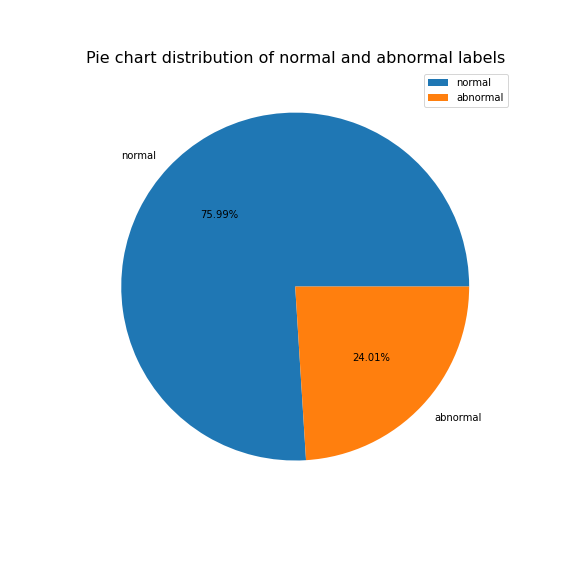#### Preparing for Multi-class Classification

A copy of DataFrame is created for Multi-class Classification.
‘attack_cat’ attribute is classified into 9 categories ‘Analysis’, ‘Backdoor’, ‘DoS’, ‘Exploits’, ‘Fuzzers’, ‘Generic’, ‘Normal’, ‘Reconnaissance’, ‘Worms’.
attack_cat is encoded using LabelEncoder(), corresponding encoded labels (0,1,2,3,4,5,6,7,8) are saved in the label attribute.
attack_cat is one-hot encoded.
Multi-class Dataset — 81173 rows, 69 columns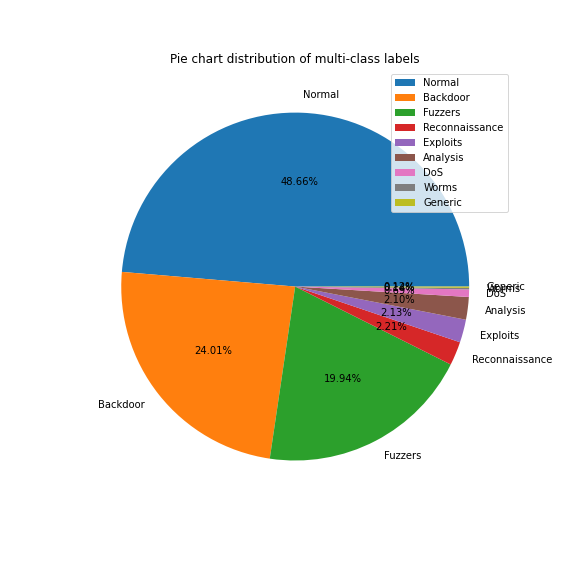#### Feature Selection

No. of attributes of ‘bin_data’ — 61
No. of attributes of ‘multi_data’ — 69
The Pearson Correlation Coefficient method is used for feature extraction.
The attributes with more than 0.3 correlation coefficient with the target attribute label were selected.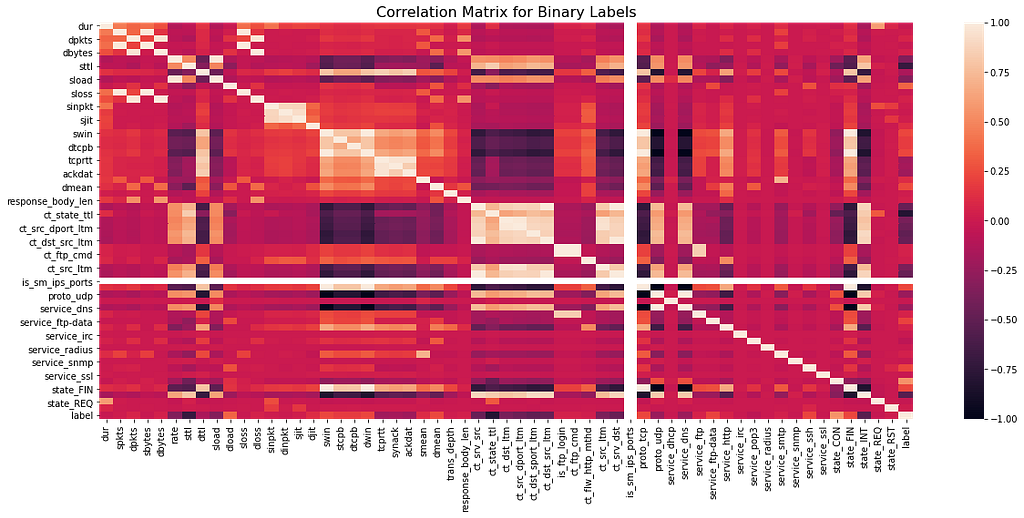No. of attributes of ‘bin_data’ after feature selection — 15
‘rate’, ‘sttl’, ‘sload’, ‘dload’, ‘ct_srv_src’, ‘ct_state_ttl’, ‘ct_dst_ltm’, ‘ct_src_dport_ltm’, ‘ct_dst_sport_ltm’, ‘ct_dst_src_ltm’, ‘ct_src_ltm’, ‘ct_srv_dst’, ‘state_CON’, ‘state_INT’, ‘label’.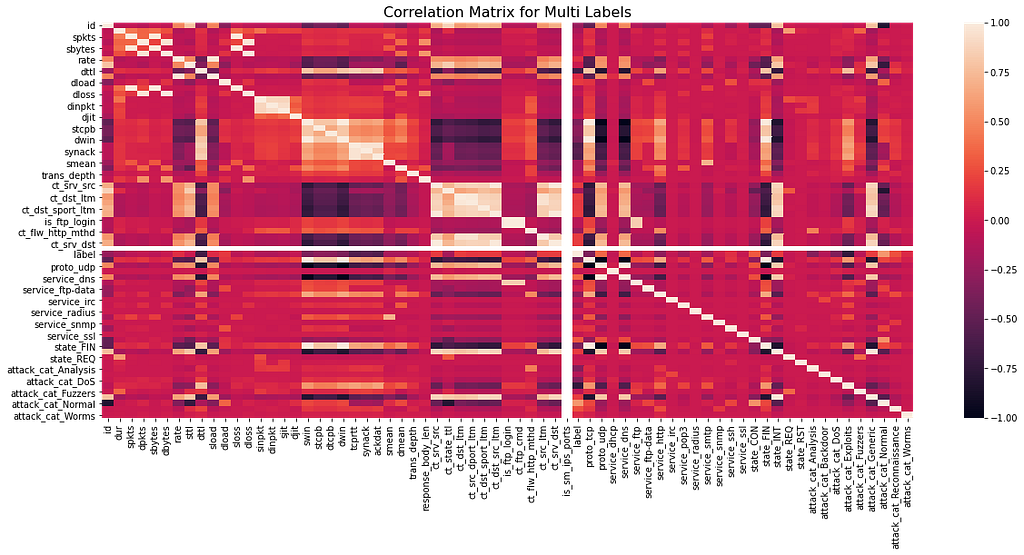No. of attributes of ‘multi_data’ after feature selection — 16
‘dttl’, ‘swin’, ‘dwin’, ‘tcprtt’, ‘synack’, ‘ackdat’, ‘label’, ‘proto_tcp’, ‘proto_udp’, ‘service_dns’, ‘state_CON’, ‘state_FIN’, ‘attack_cat_Analysis’, ‘attack_cat_DoS’, ‘attack_cat_Exploits’, ‘attack_cat_Normal’.

### Splitting Dataset into Training and Testing

Randomly splitting the bin_data in 80% for training and 20% for testing.
Randomly splitting the multi_data in 70% for training and 30% for testing.
Target feature — label

### Decision Tree Classifier

#### Binary Classification

Accuracy — 98.09054511857099
Mean Absolute Error — 0.019094548814290114
Mean Squared Error — 0.019094548814290114
Root Mean Squared Error — 0.13818302650575473
R2 Score — 89.55757103838098

DecisionTreeClassifier(ccp_alpha=0.0, class_weight=None, criterion=’gini’, max_depth=None, max_features=None, max_leaf_nodes=None, min_impurity_decrease=0.0, min_impurity_split=None, min_samples_leaf=1, min_samples_split=2, min_weight_fraction_leaf=0.0, presort=’deprecated’, random_state=123, splitter=’best’)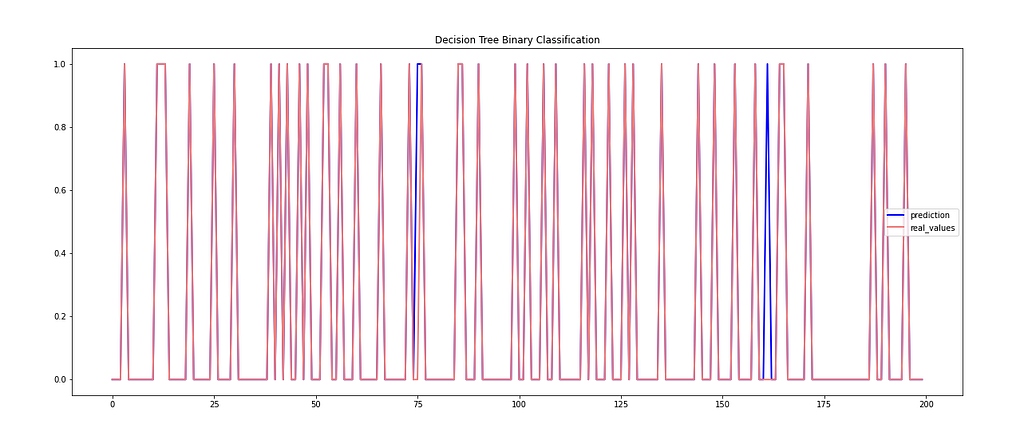Binary Classification with Decision Tree Classifier

#### Multi-class Classification

Accuracy — 97.19940867279895
Mean Absolute Error — 0.06800262812089355
Mean Squared Error — 0.20532194480946123
Root Mean Squared Error — 0.4531246459965086
R2 Score — 86.17743099336013

DecisionTreeClassifier(ccp_alpha=0.0, class_weight=None, criterion=’gini’, max_depth=None, max_features=None, max_leaf_nodes=None, min_impurity_decrease=0.0, min_impurity_split=None, min_samples_leaf=1, min_samples_split=2, min_weight_fraction_leaf=0.0, presort=’deprecated’, random_state=123, splitter=’best’)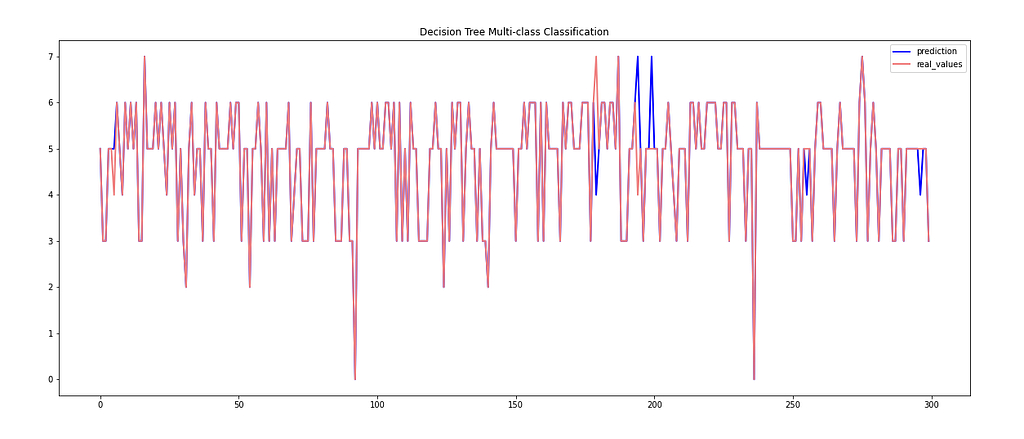Multi-class Classification with Decision Tree Classifier

### K-Nearest Neighbor Classifier

#### Binary Classification

Accuracy — 98.3061287342162
Mean Absolute Error — 0.016938712657838004
Mean Squared Error — 0.016938712657838004
Root Mean Squared Error — 0.13014880966738807
R2 Score — 90.74435871039374

KNeighborsClassifier(algorithm=’auto’, leaf_size=30, metric=’minkowski’, metric_params=None, n_jobs=None, n_neighbors=5, p=2, weights=’uniform’)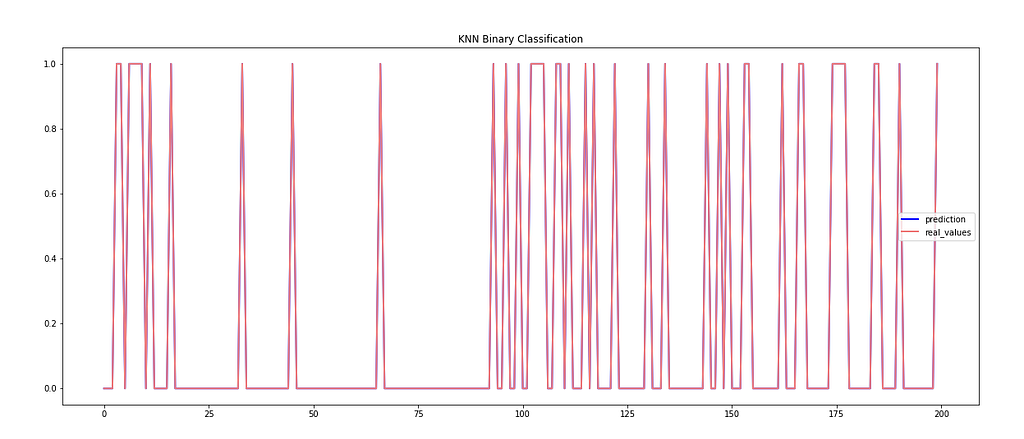Binary Classification with KNN Classifier

#### Multi-class Classification

Accuracy — 97.36777266754271
Mean Absolute Error — 0.06508705650459921
Mean Squared Error — 0.19411136662286466
Root Mean Squared Error — 0.44058071521897624
R2 Score — 86.92848100772136

KNeighborsClassifier(algorithm=’auto’, leaf_size=30, metric=’minkowski’, metric_params=None, n_jobs=None, n_neighbors=5, p=2, weights=’uniform’)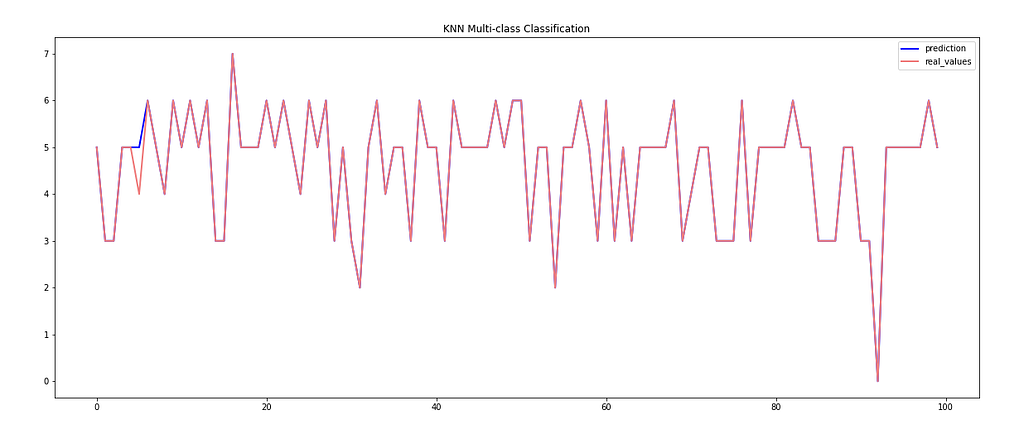Multi-class Classification with KNN Classifier

### Linear Regression Model

#### Binary Classification

Accuracy — 97.80720665229443
Mean Absolute Error — 0.021927933477055742
Mean Squared Error — 0.021927933477055742
Root Mean Squared Error — 0.1480808342664767
R2 Score — 88.20923868071647

LinearRegression(copy_X=True, fit_intercept=True, n_jobs=None, normalize=False)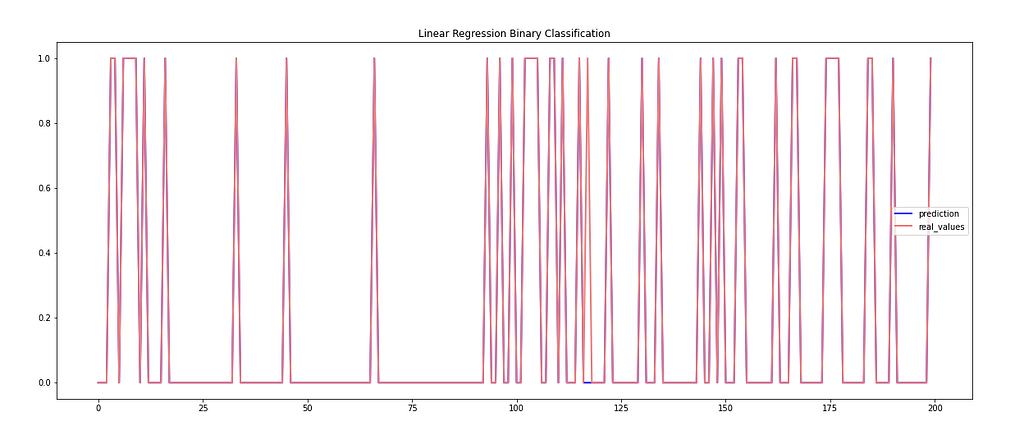Binary Classification with Linear Regression Model

#### Multi-class Classification

Accuracy — 95.12976346911958
Mean Absolute Error — 0.06824901445466491
Mean Squared Error — 0.12146846254927726
Root Mean Squared Error — 0.3485232596962178
R2 Score — 91.82055676180129

LinearRegression(copy_X=True, fit_intercept=True, n_jobs=None, normalize=False)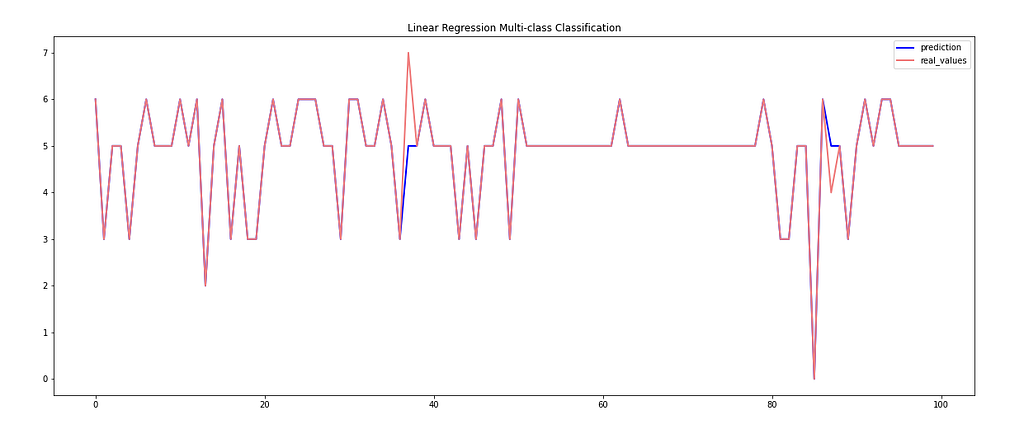Multi-class Classification with Linear Regression Model

### Linear Support Vector Machine

#### Binary Classification

Accuracy — 97.85032337542347
Mean Absolute Error — 0.021496766245765322
Mean Squared Error — 0.021496766245765322
Root Mean Squared Error — 0.1466177555610688
R2 Score — 88.45167193436498

SVC(C=1.0, break_ties=False, cache_size=200, class_weight=None, coef0=0.0, decision_function_shape=’ovr’, degree=3, gamma=’auto’, kernel=’linear’, max_iter=-1, probability=False, random_state=None, shrinking=True, tol=0.001, verbose=False)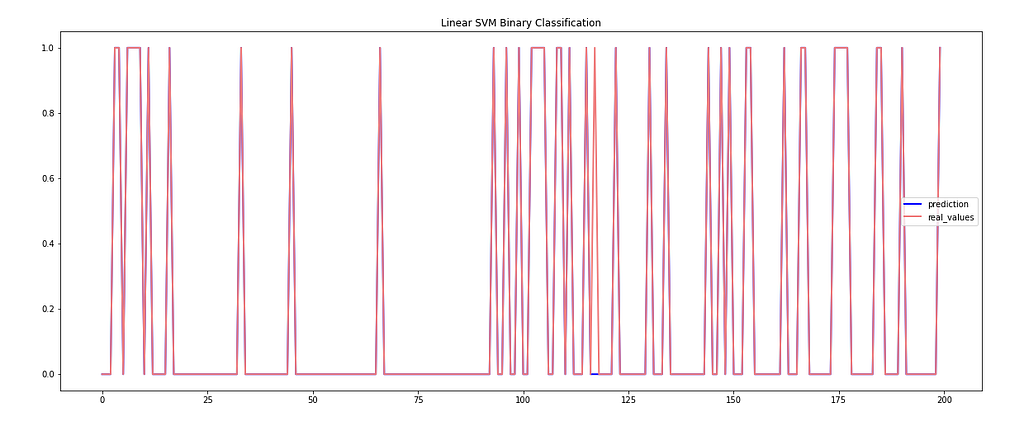Binary Classification with Linear Support Vector Machine

#### Multi-class Classification

Accuracy — 97.59362680683311
Mean Absolute Error — 0.059912943495400786
Mean Squared Error — 0.17941031537450722
Root Mean Squared Error — 0.42356854861345317
R2 Score — 87.93449282205455

SVC(C=1.0, break_ties=False, cache_size=200, class_weight=None, coef0=0.0, decision_function_shape=’ovr’, degree=3, gamma=’auto’, kernel=’linear’, max_iter=-1, probability=False, random_state=None, shrinking=True, tol=0.001, verbose=False)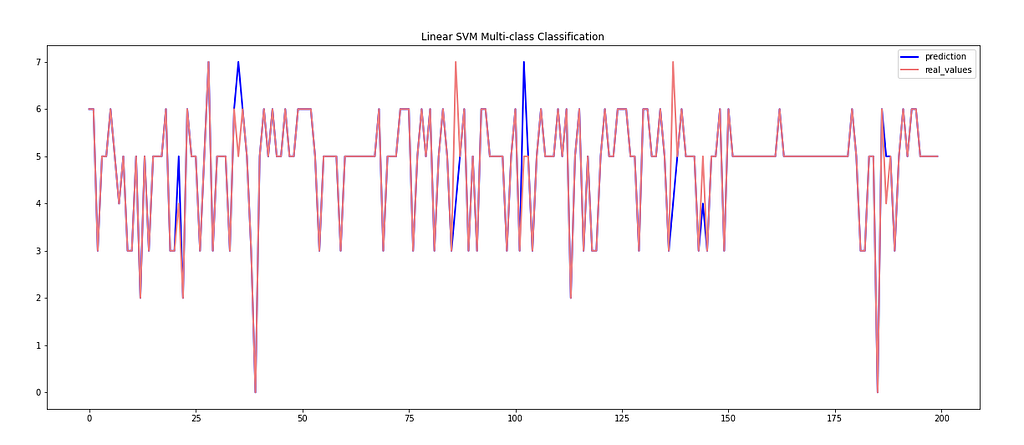Multi-class Classification with Linear Support Vector Machine

### Logistic Regression Model

#### Binary Classification

Accuracy — 97.80104712041884
Mean Absolute Error — 0.02198952879581152
Mean Squared Error — 0.02198952879581152
Root Mean Squared Error — 0.1482886671186019
R2 Score — 88.17947258428785

LogisticRegression(C=1.0, class_weight=None, dual=False, fit_intercept=True, intercept_scaling=1, l1_ratio=None, max_iter=5000, multi_class=’auto’, n_jobs=None, penalty=’l2′, random_state=123, solver=’lbfgs’, tol=0.0001, verbose=0, warm_start=False)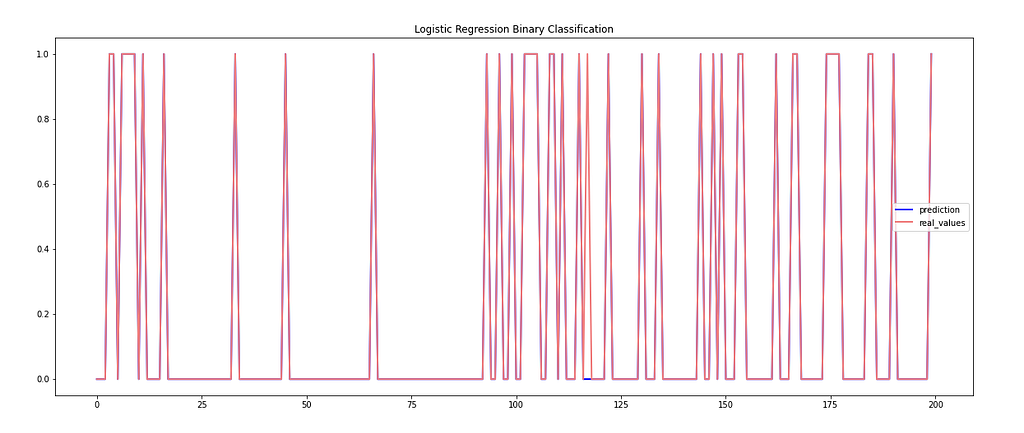Binary Classification with Logistic Regression Model

#### Multi-class Classification

Accuracy — 97.58952036793693
Mean Absolute Error — 0.060077201051248356
Mean Squared Error — 0.18056011826544022
Root Mean Squared Error — 0.42492366169165047
R2 Score — 87.87674567880146

LogisticRegression(C=1.0, class_weight=None, dual=False, fit_intercept=True, intercept_scaling=1, l1_ratio=None, max_iter=5000, multi_class=’multinomial’, n_jobs=None, penalty=’l2′, random_state=123, solver=’newton-cg’, tol=0.0001, verbose=0, warm_start=False)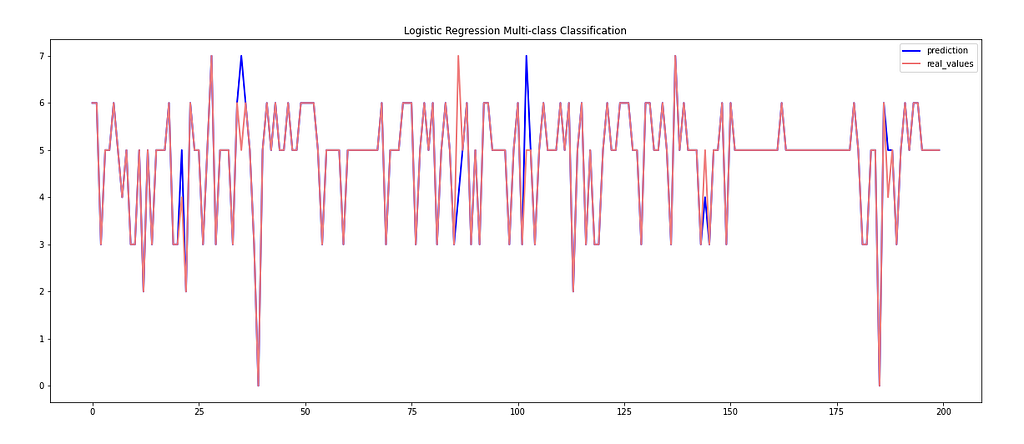Multi-class Classification with Logistic Regression Model

### Multi-Layer Perceptron Classifier

#### Binary Classification

Accuracy — 98.36772405297197
Mean Absolute Error — 0.01632275947028026
Mean Squared Error — 0.01632275947028026
Root Mean Squared Error — 0.12776055522061674
R2 Score — 91.10646238100463

MLPClassifier(activation=’relu’, alpha=0.0001, batch_size=’auto’, beta_1=0.9, beta_2=0.999, early_stopping=False, epsilon=1e-08, hidden_layer_sizes=(100,), learning_rate=’constant’, learning_rate_init=0.001, max_fun=15000, max_iter=8000, momentum=0.9, n_iter_no_change=10, nesterovs_momentum=True, power_t=0.5, random_state=123, shuffle=True, solver=’adam’, tol=0.0001, validation_fraction=0.1, verbose=False, warm_start=False)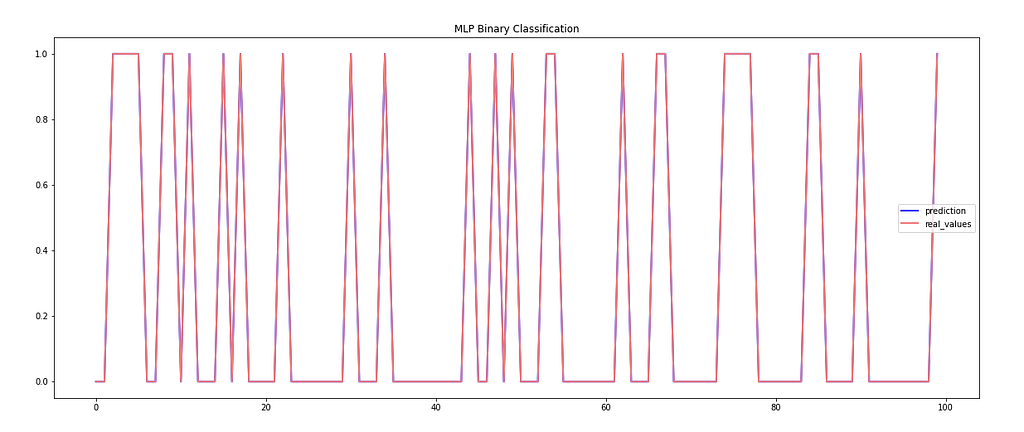Binary Classification with Multi-Layer Perceptron Classifier

#### Multi-class Classification

Accuracy — 97.54434954007884
Mean Absolute Error — 0.06065210249671485
Mean Squared Error — 0.17858902759526937
Root Mean Squared Error — 0.4225979502970517
R2 Score — 87.97913543550516

MLPClassifier(activation=’relu’, alpha=0.0001, batch_size=’auto’, beta_1=0.9, beta_2=0.999, early_stopping=False, epsilon=1e-08, hidden_layer_sizes=(100,), learning_rate=’constant’, learning_rate_init=0.001, max_fun=15000, max_iter=8000, momentum=0.9, n_iter_no_change=10, nesterovs_momentum=True, power_t=0.5, random_state=123, shuffle=True, solver=’adam’, tol=0.0001, validation_fraction=0.1, verbose=False, warm_start=False)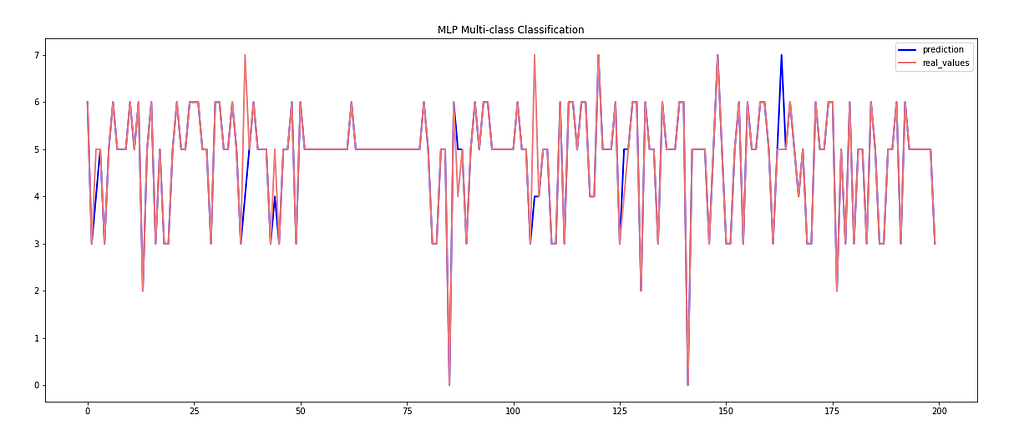Multi-class Classification with Multi-Layer Perceptron Classifier

### Random Forest Classifier

#### Binary Classification

Accuracy — 98.64490298737296
Mean Absolute Error — 0.013550970126270403
Mean Squared Error — 0.013550970126270403
Root Mean Squared Error — 0.1164086342427846
R2 Score — 92.59509512345335

RandomForestClassifier(bootstrap=True, ccp_alpha=0.0, class_weight=None, criterion=’gini’, max_depth=None, max_features=’auto’, max_leaf_nodes=None, max_samples=None, min_impurity_decrease=0.0, min_impurity_split=None, min_samples_leaf=1, min_samples_split=2, min_weight_fraction_leaf=0.0, n_estimators=100, n_jobs=None, oob_score=False, random_state=123, verbose=0, warm_start=False)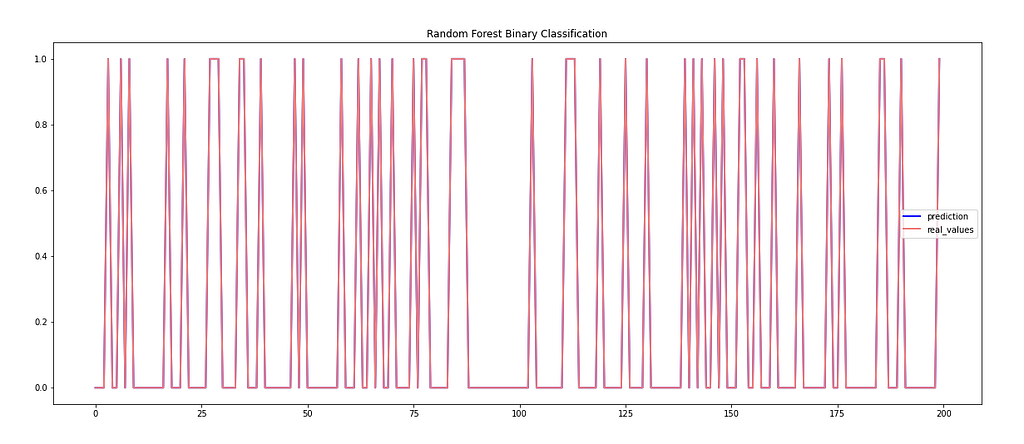Binary Classification with Random Forest Classifier

#### Multi-class Classification

Accuracy — 97.31849540078844
Mean Absolute Error — 0.06611366622864652
Mean Squared Error — 0.1985052562417871
Root Mean Squared Error — 0.4455392869790352
R2 Score — 86.6379909424011

RandomForestClassifier(bootstrap=True, ccp_alpha=0.0, class_weight=None, criterion=’gini’, max_depth=None, max_features=’auto’, max_leaf_nodes=None, max_samples=None, min_impurity_decrease=0.0, min_impurity_split=None, min_samples_leaf=1, min_samples_split=2, min_weight_fraction_leaf=0.0, n_estimators=100, n_jobs=None, oob_score=False, random_state=50, verbose=0, warm_start=False)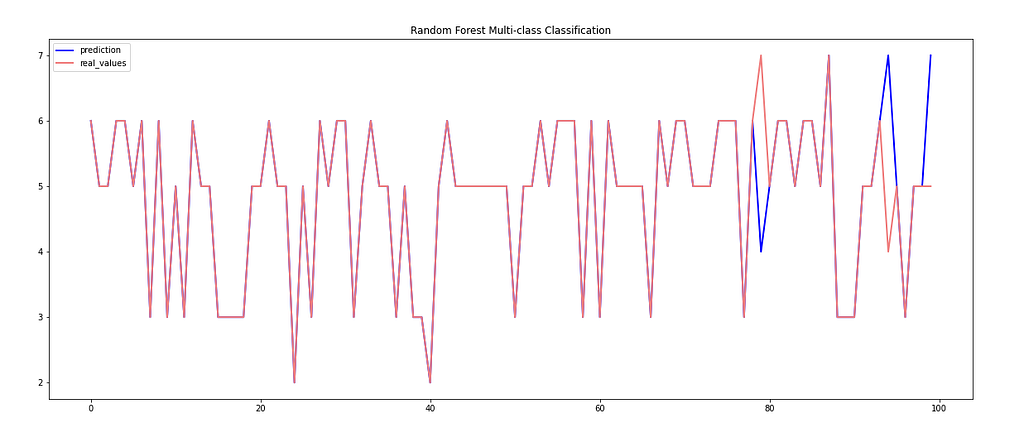Multi-class Classification with Random Forest Classifier

### Get the complete code, models, plots on my GitHub account

GitHub – abhinav-bhardwaj/IoT-Network-Intrusion-Detection-System-UNSW-NB15: Network Intrusion Detection based on various machine learning and deep learning algorithms using UNSW-NB15 Dataset

### Citations

N. Moustafa and J. Slay, “UNSW-NB15: a comprehensive data set for network intrusion detection systems (UNSW-NB15 network data set),” 2015 Military Communications and Information Systems Conference (MilCIS), 2015, pp. 1–6, DOI: 10.1109/MilCIS.2015.7348942
Nour Moustafa & Jill Slay (2016) The evaluation of Network Anomaly Detection Systems: Statistical analysis of the UNSW-NB15 data set and the comparison with the KDD99 data set, Information Security Journal: A Global Perspective, 25:1–3, 18–31, DOI: 10.1080/19393555.2015.1125974Cyberattacks Detection in IoT-based Smart City Network Traffic was originally published in Towards AI on Medium, where people are continuing the conversation by highlighting and responding to this story.

Published via Towards AI